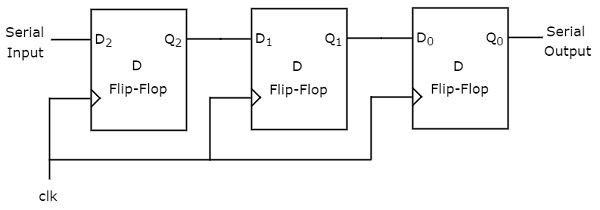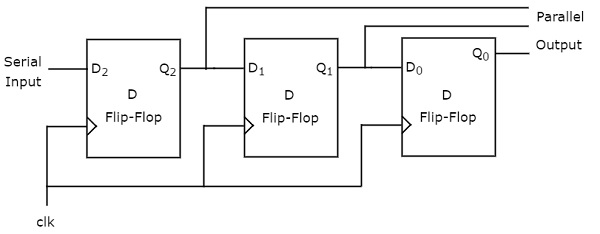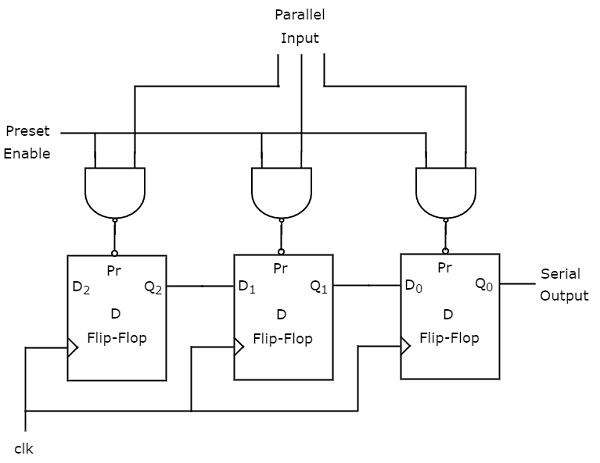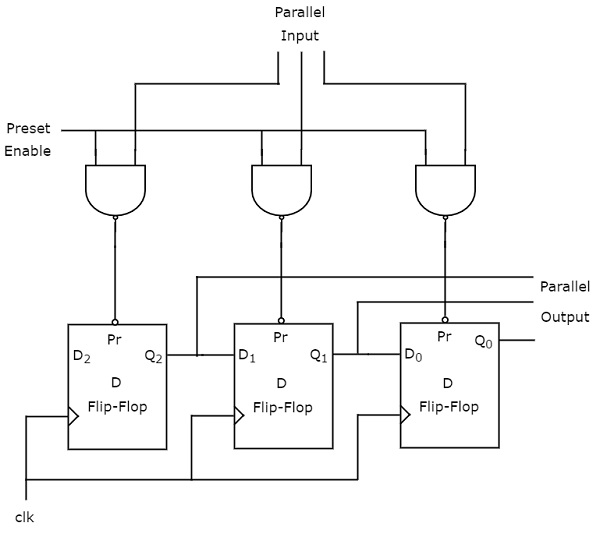# Digital Circuits - Shift Registers

We know that one flip-flop can store one-bit of information. In order to store multiple bits of information, we require multiple flip-flops. The group of flip-flops, which are used to hold (store) the binary data is known as register.

If the register is capable of shifting bits either towards right hand side or towards left hand side is known as shift register. An ‘N’ bit shift register contains ‘N’ flip-flops. Following are the four types of shift registers based on applying inputs and accessing of outputs.

• Serial In − Serial Out shift register
• Serial In − Parallel Out shift register
• Parallel In − Serial Out shift register
• Parallel In − Parallel Out shift register

## Serial In − Serial Out (SISO) Shift Register

The shift register, which allows serial input and produces serial output is known as Serial In – Serial Out (SISO) shift register. The block diagram of 3-bit SISO shift register is shown in the following figure.This block diagram consists of three D flip-flops, which are cascaded. That means, output of one D flip-flop is connected as the input of next D flip-flop. All these flip-flops are synchronous with each other since, the same clock signal is applied to each one.

In this shift register, we can send the bits serially from the input of left most D flip-flop. Hence, this input is also called as serial input. For every positive edge triggering of clock signal, the data shifts from one stage to the next. So, we can receive the bits serially from the output of right most D flip-flop. Hence, this output is also called as serial output.

### Example

Let us see the working of 3-bit SISO shift register by sending the binary information “011” from LSB to MSB serially at the input.

Assume, initial status of the D flip-flops from leftmost to rightmost is $Q_{2}Q_{1}Q_{0}=000$. We can understand the working of 3-bit SISO shift register from the following table.

No of positive edge of Clock Serial Input Q2 Q1 Q0
0 - 0 0 0
1 1(LSB) 1 0 0
2 1 1 1 0
3 0(MSB) 0 1 1(LSB)
4 - - 0 1
5 - - - 0(MSB)

The initial status of the D flip-flops in the absence of clock signal is $Q_{2}Q_{1}Q_{0}=000$. Here, the serial output is coming from $Q_{0}$. So, the LSB (1) is received at 3rd positive edge of clock and the MSB (0) is received at 5th positive edge of clock.

Therefore, the 3-bit SISO shift register requires five clock pulses in order to produce the valid output. Similarly, the N-bit SISO shift register requires 2N-1 clock pulses in order to shift ‘N’ bit information.

## Serial In - Parallel Out (SIPO) Shift Register

The shift register, which allows serial input and produces parallel output is known as Serial In – Parallel Out (SIPO) shift register. The block diagram of 3-bit SIPO shift register is shown in the following figure.This circuit consists of three D flip-flops, which are cascaded. That means, output of one D flip-flop is connected as the input of next D flip-flop. All these flip-flops are synchronous with each other since, the same clock signal is applied to each one.

In this shift register, we can send the bits serially from the input of left most D flip-flop. Hence, this input is also called as serial input. For every positive edge triggering of clock signal, the data shifts from one stage to the next. In this case, we can access the outputs of each D flip-flop in parallel. So, we will get parallel outputs from this shift register.

### Example

Let us see the working of 3-bit SIPO shift register by sending the binary information “011” from LSB to MSB serially at the input.

Assume, initial status of the D flip-flops from leftmost to rightmost is $Q_{2}Q_{1}Q_{0}=000$. Here, $Q_{2}$ & $Q_{0}$ are MSB & LSB respectively. We can understand the working of 3-bit SIPO shift register from the following table.

No of positive edge of Clock Serial Input Q2(MSB) Q1 Q0(LSB)
0 - 0 0 0
1 1(LSB) 1 0 0
2 1 1 1 0
3 0(MSB) 0 1 1

The initial status of the D flip-flops in the absence of clock signal is $Q_{2}Q_{1}Q_{0}=000$. The binary information “011” is obtained in parallel at the outputs of D flip-flops for third positive edge of clock.

So, the 3-bit SIPO shift register requires three clock pulses in order to produce the valid output. Similarly, the N-bit SIPO shift register requires N clock pulses in order to shift ‘N’ bit information.

## Parallel In − Serial Out (PISO) Shift Register

The shift register, which allows parallel input and produces serial output is known as Parallel In − Serial Out (PISO) shift register. The block diagram of 3-bit PISO shift register is shown in the following figure.This circuit consists of three D flip-flops, which are cascaded. That means, output of one D flip-flop is connected as the input of next D flip-flop. All these flip-flops are synchronous with each other since, the same clock signal is applied to each one.

In this shift register, we can apply the parallel inputs to each D flip-flop by making Preset Enable to 1. For every positive edge triggering of clock signal, the data shifts from one stage to the next. So, we will get the serial output from the right most D flip-flop.

### Example

Let us see the working of 3-bit PISO shift register by applying the binary information “011” in parallel through preset inputs.

Since the preset inputs are applied before positive edge of Clock, the initial status of the D flip-flops from leftmost to rightmost will be $Q_{2}Q_{1}Q_{0}=011$. We can understand the working of 3-bit PISO shift register from the following table.

No of positive edge of Clock Q2 Q1 Q0
0 0 1 1(LSB)
1 - 0 1
2 - - 0(LSB)

Here, the serial output is coming from $Q_{0}$. So, the LSB (1) is received before applying positive edge of clock and the MSB (0) is received at 2nd positive edge of clock.

Therefore, the 3-bit PISO shift register requires two clock pulses in order to produce the valid output. Similarly, the N-bit PISO shift register requires N-1 clock pulses in order to shift ‘N’ bit information.

## Parallel In - Parallel Out (PIPO) Shift Register

The shift register, which allows parallel input and produces parallel output is known as Parallel In − Parallel Out (PIPO) shift register. The block diagram of 3-bit PIPO shift register is shown in the following figure.This circuit consists of three D flip-flops, which are cascaded. That means, output of one D flip-flop is connected as the input of next D flip-flop. All these flip-flops are synchronous with each other since, the same clock signal is applied to each one.

In this shift register, we can apply the parallel inputs to each D flip-flop by making Preset Enable to 1. We can apply the parallel inputs through preset or clear. These two are asynchronous inputs. That means, the flip-flops produce the corresponding outputs, based on the values of asynchronous inputs. In this case, the effect of outputs is independent of clock transition. So, we will get the parallel outputs from each D flip-flop.

### Example

Let us see the working of 3-bit PIPO shift register by applying the binary information “011” in parallel through preset inputs.

Since the preset inputs are applied before positive edge of Clock, the initial status of the D flip-flops from leftmost to rightmost will be $Q_{2}Q_{1}Q_{0}=011$. So, the binary information “011” is obtained in parallel at the outputs of D flip-flops before applying positive edge of clock.

Therefore, the 3-bit PIPO shift register requires zero clock pulses in order to produce the valid output. Similarly, the N-bit PIPO shift register doesn’t require any clock pulse in order to shift ‘N’ bit information.